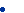InvestmentTools.com   |     homeMany shall be restored that are now fallen and many Shall fall that are now in honor. HoraceBenjamin Graham's 10 RulesMore MiscellaneousThe 10 Ben Graham Rules:1) An earnings-to-price yield of twice the triple-A bond. If the triple-A bond yield is, say 8%, then the required earnings yield is 16%. In reciprocal form, that's a price/earnings ratio of 6.25. 2) A P/E ratio down to four-tenth of the highest average P/E ratio the stock attained in the most recent five years. ( Average P/E ratio was defined as average stock price for a given year divided by the earnings for that year.) 3) A dividend yield of two-thirds the triple-A bond yield. 4) A stock price down to two-thirds of tangible book value per share. 5) A stock price down to two-thirds of "net current asset value" or "net quick liquidation value." This figure is defined as current assets less total debt. Fixed assets are not included. 6) Total debt less than tangible book value. 7) Current ratio ( current assets divided by current liabilities) of two or more. 8) Total debt equal or less than twice the net quick liquidation value as defined in No.5. 9) Earnings growth over the most recent ten years of 7% compounded - that is a doubling of earnings in a ten-year period. 10) Stability of growth in earnings, defined as no more then two declines of 5% or greater in year-end earnings (relative to the previous year) in the most recent ten years.